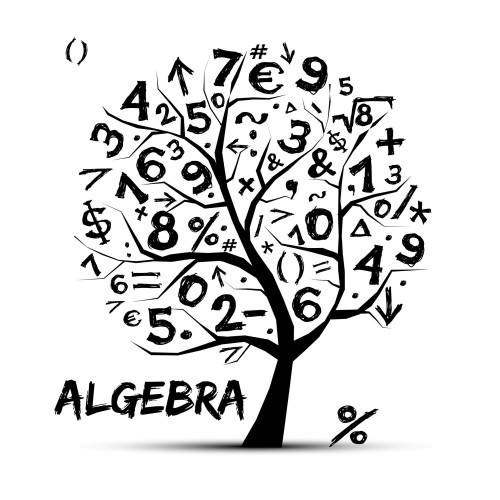This free e-book was created withIt's simple and free.

# What is Algebra?

• Joined Feb 2016
• Published Books 1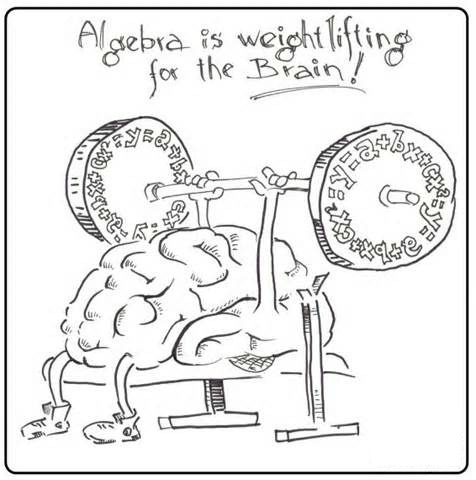# What is Algebra?

Algebra is a branch of mathematics that not only uses numbers, but letters that represent unknown numbers.

3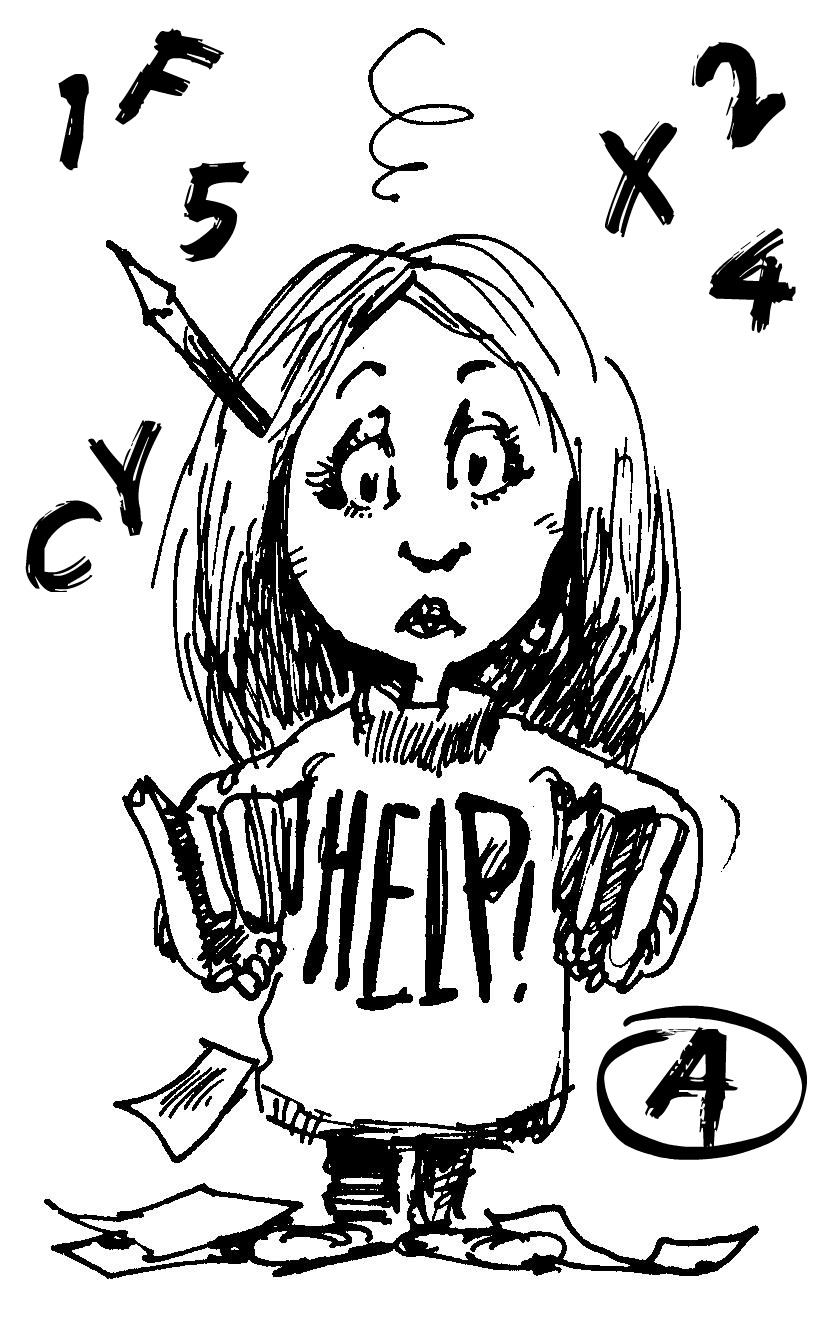Any letter can represent an unknown number in an algebraic equation; however, the most popular letters or variables are x, y and z.

So how do you solve an algebraic equation for its unknown variable?

You must find the number that your variable stands for that will make both sides of the equation equal to each other.

For example, you have the equation 6 + x = 9

We need to find a number (x) that when added to 6 will equal 9.

We know that: 6 + 3 = 9

So this tells us that x = 3

5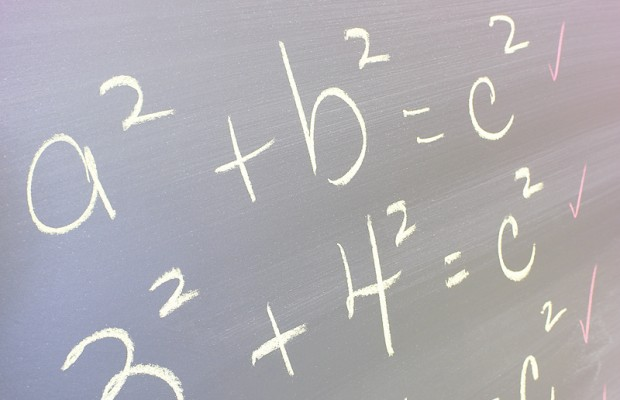*A specific variable can only stand for ONE specific number.

*There can be multiple variables in an equation, but they must stand for different numbers.

For example, you have the equation x + x = 12

Even though there are several possibilities (8 + 4 or 10 + 2 or 3 +9, etc.), x can only equal one number. So in this case, x could only be 6.

6 + 6 = 12

For this example, if you wanted to use two different numbers such as 8 + 4, then your equation would have to have two different variables. Like this: x + y = 12 where x = 8 and y = 4

8 + 4 = 12

7# Multiplication is Very Important!

Multiplication is known as the default or implied application in Algebra.

This means that if there isn’t an operation between two variables or a variable and a number, you must multiply them together.

For example, if you have the equation yz = 24 since the y and the z are right next to each other, it means the same as y x z = 24

Writing it in this form makes your equation less confusing, especially if you have x as your variable.

You can also use parenthesis () to signify multiplication.

For example: y(z) = 24 or x(y + z) = 24 or (xy)(z + x) = 24 ect.

9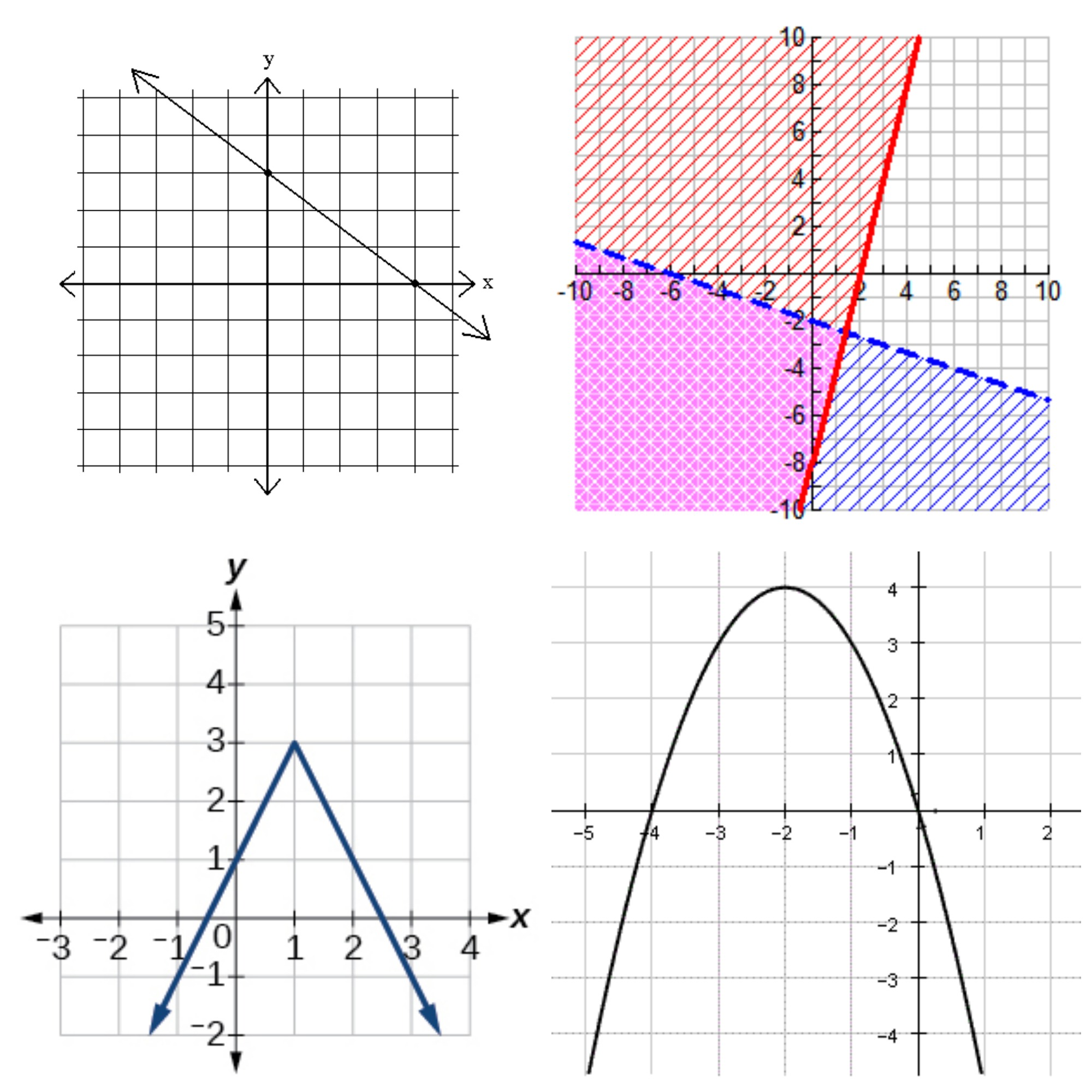# Graphing

You will also graph equations on the x and y plane in Algebra.

Some types of equations are: linear equations, quadratic equations, inequalities, absolute value, and much more!

11

The following website contains Algebra facts, definitions, practice problems, games, and much more!

Math is Fun! Introduction to Algebra

12

Math Antics provides various instructional videos on different types of math. Watch the video “Algebra Basics: What Is Algebra?” below for more insight into the world of Algebra!

Algebra Basics: What Is Algebra?

13
This free e-book was created withSkip to content